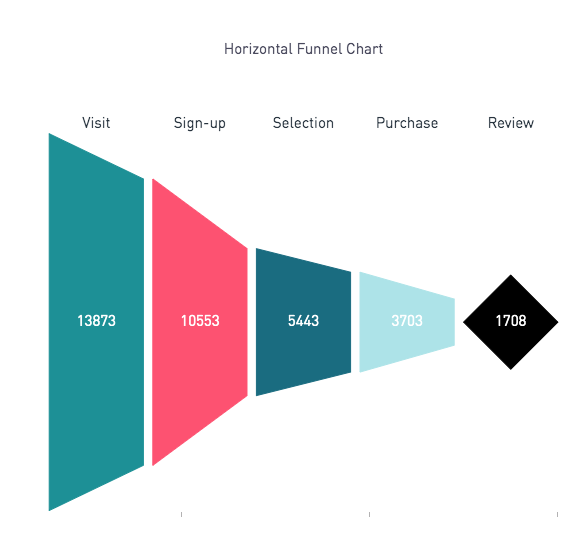Horizontal Funnel Chart — Plot.lyVisually it can be preferable to display a funnel chart horizontally. The following code outlines how to do this.  Note, your SQL output must have 2 columns titled as follows:

1. phases: the names of each stage along the funnel
2. values: the value associated with each stage along the funnel

If the data is in the incorrect format, dummy data will show in the funnel, matching the image above.

Here is the Python 3.6 script used to generate the funnel chart:

# Resource: Based off of this tutorial: https://moderndata.plot.ly/funnel-charts-in-python-using-plotly/

# PERISCOPE HORIZONTAL FUNNEL TEMPLATE
# SQL output should have 2 columns:
#    1) phases: the names of each stage along the funnel
#    2) values: the value associated with each stage along the funnel

# Import libraries
import pandas as pd
import plotly.plotly as py
import plotly.graph_objs as go

# Create Dummy Data
phases = ['Visit', 'Sign-up', 'Selection', 'Purchase', 'Review']
values = [13873, 10553, 5443, 3703, 1708]
data = {'phases':phases, 'values':values}
dummy_df = pd.DataFrame(data)

# HELPER FUNCTION: For annotation text
style = ';'.join([f'{key.replace("_","-")}:{settings[key]}' for key in settings])
return f'<a href="{link}" style="{style}">{text}</a>'

# MAIN FUNCTION: input a dataframe
def plot(df, annotation = None):
phases = df['phases']
values = df['values']

# Colors of funnel stages. UPDATE to include more rgb triplets if there are over 5 steps in the funnel
colors = ['rgb(32,155,160)', 'rgb(253,93,124)', 'rgb(28,119,139)', 'rgb(182,231,235)', 'rgb(0,0,0)']

n_phase = len(phases)

# the fixed height for the plot
plot_height = 400

# width of a section and difference between sections
section_w = 100
section_d = 10

# multiply factor to calculate the width of other sections
unit_height = plot_height / max(values)

# height for all the sections (phases)
phase_h = [int(value * unit_height) for value in values]
width = section_w * n_phase + section_d * (n_phase-1)

# Initiating variables
shapes = []
label_x = []

# Creating the shapes to create the funnel
for i in range(n_phase):
# Setup for creating a diamond to show the last stage of the funnel
if (i == n_phase-1):
points = [phase_h[i]/2, width, phase_h[i]/2, width - section_w]
max_final = points
midpoint_final = max_final / 2
path = 'M -{0} 0 L -{1} {1} L 0 0 L -{1} -{1} Z'.format(max_final, midpoint_final)
# Setup for creating a trapezoid for all other stages of the funnel
else:
points = [phase_h[i]/2, width, phase_h[i+1]/2, width - section_w]
path = 'M -{1} {0} L -{3} {2} L -{3} -{2} L -{1} -{0} Z'.format(*points)

# Create the shapes
shape = {
'type': 'path',
'path': path,
'fillcolor': colors[i],
'layer': 'below',
'line': {
'width': 1,
'color': colors[i]
}
}
shapes.append(shape)

# Y-axis location for this section's details (phase name and value)
label_x.append((width - (section_w / 2))*-1)
width = width - (section_w + section_d)

# For phase names
label_trace = go.Scatter(
x=label_x,
y=*n_phase,
mode='text',
text=phases,
textfont=dict(
color='rgba(44,58,71,1)',
size=15
)
)

# For phase values
value_trace = go.Scatter(
x = label_x,
y = *n_phase,
mode='text',
text=values,
textfont=dict(
color='rgba(256,256,256,1)',
size=15
)
)

# Bring label names and amounts together in a data parameter
data = [label_trace, value_trace]

# Add layout parameters
layout = go.Layout(
title='Horizontal Funnel Chart',
shapes=shapes,
height=800,
width=560,
showlegend=False,
xaxis=dict(
showticklabels=False,
zeroline=False,
showgrid = False
),
yaxis=dict(
showticklabels=False,
zeroline=False,
showgrid = False,
scaleanchor="x",
scaleratio=1
)
)

# Plot the figure
fig = go.Figure(data = data, layout=layout)
periscope.plotly(fig)

# We try to to plot the SQL output. If it is not in the correct format, the dummy data will display. See stdout tab for the error message.
try:
plot(df)
except Exception as e:
print(e)
plot(dummy_df)

Source: Adapted from the tutorial here